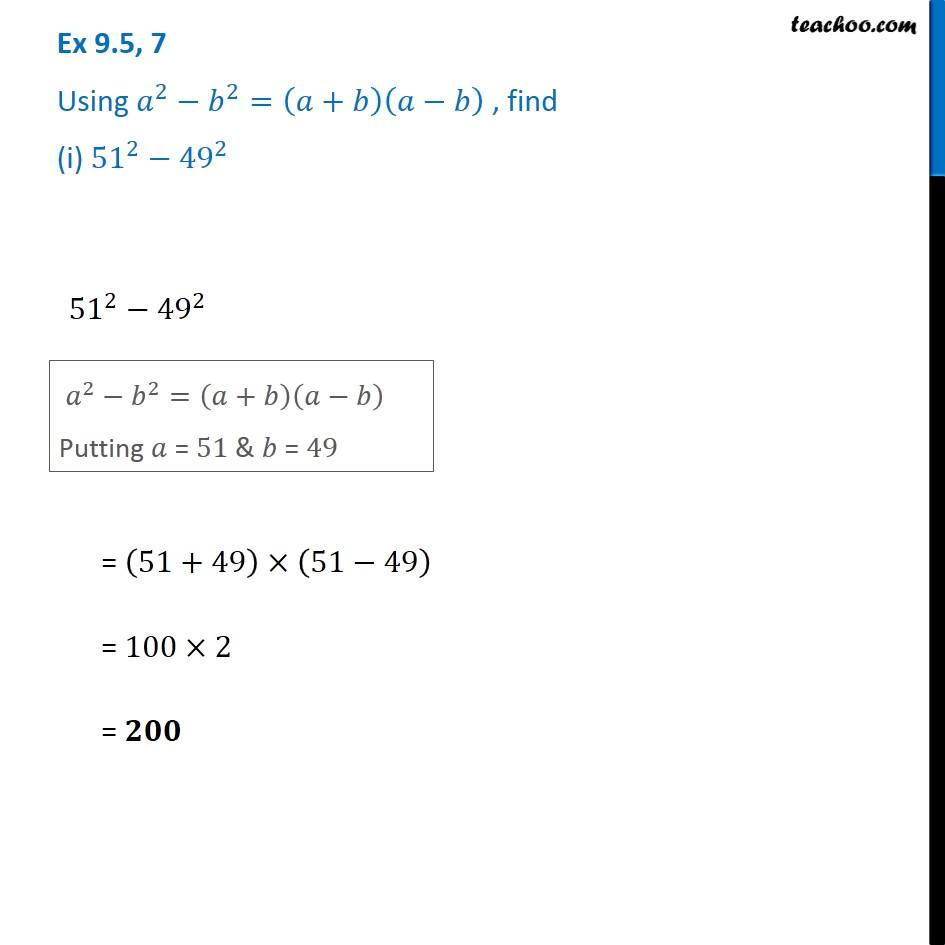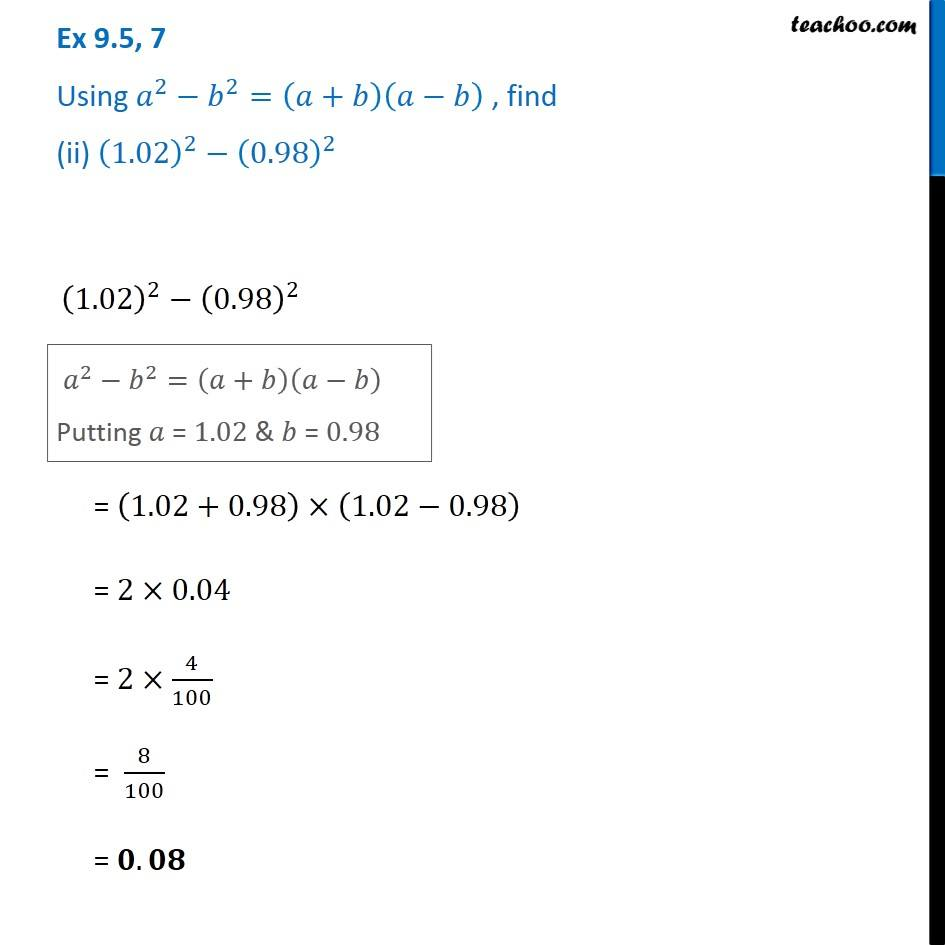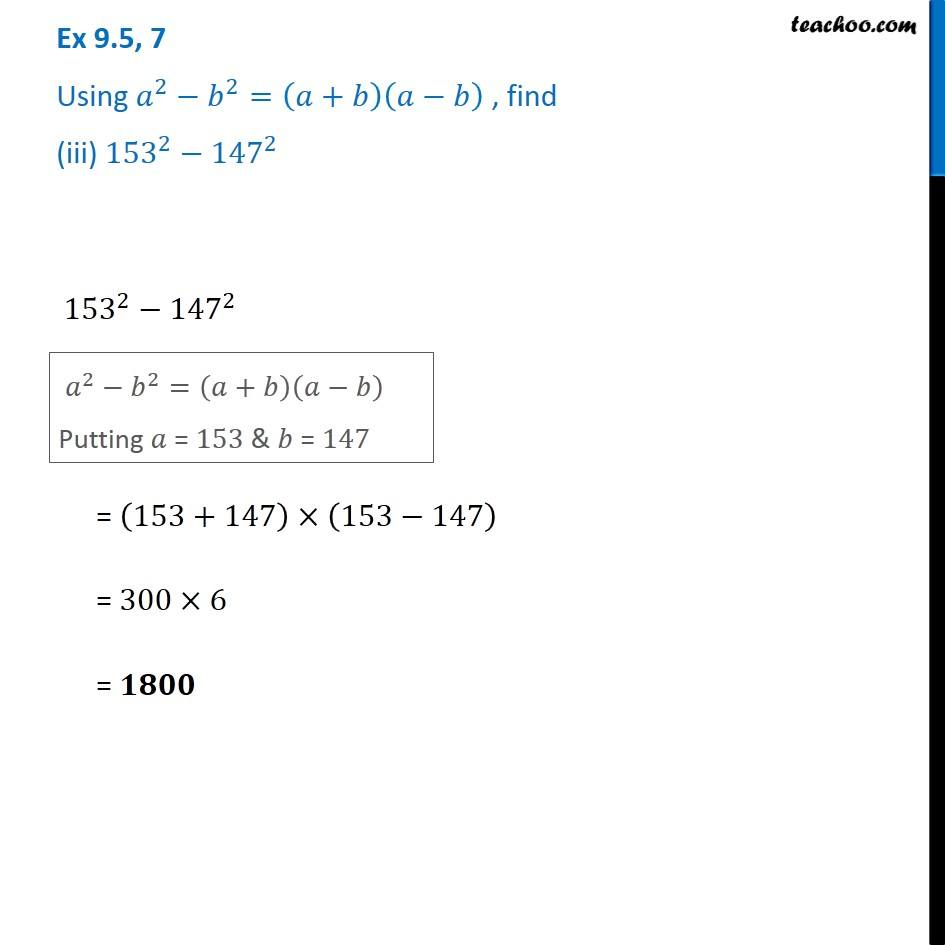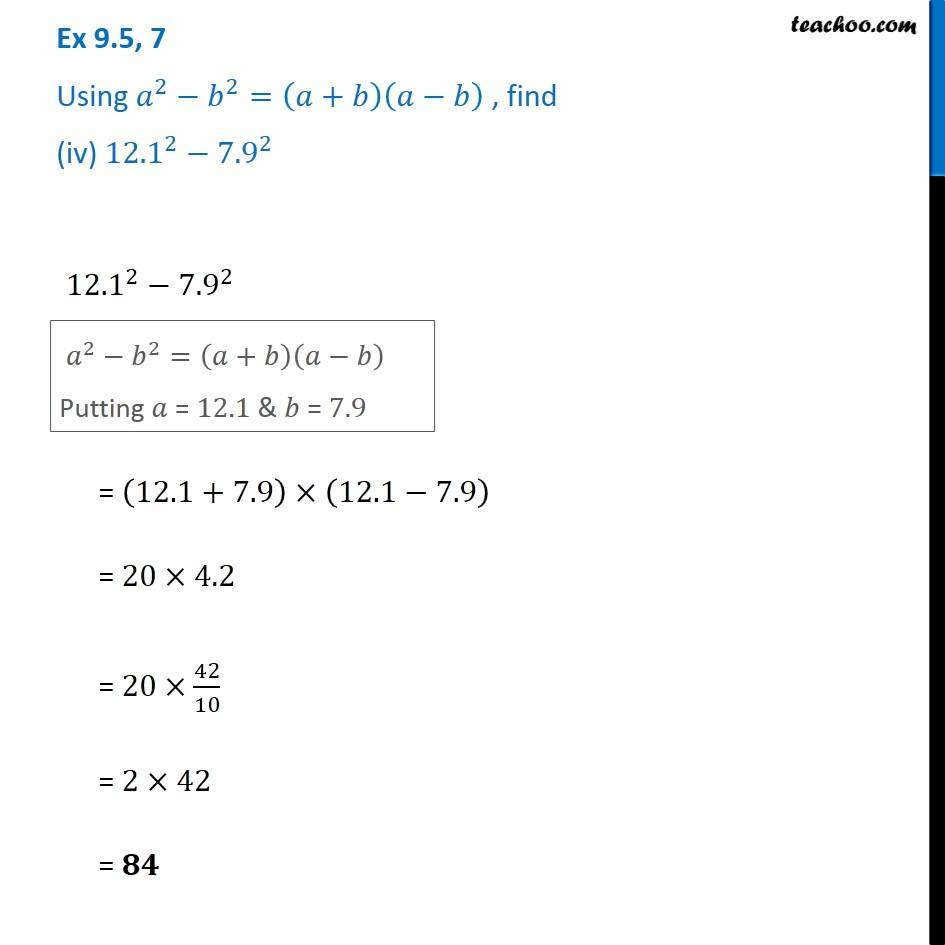1. Chapter 9 Class 8 Algebraic Expressions and Identities
2. Serial order wise
3. Ex 9.5

Transcript

Ex 9.5, 7 Using 𝑎^2−𝑏^2=(𝑎+𝑏)(𝑎−𝑏) , find (i) 〖51〗^2−〖49〗^2 〖51〗^2−〖49〗^2 𝑎^2−𝑏^2=(𝑎+𝑏)(𝑎−𝑏) Putting 𝑎 = 51 & 𝑏 = 49 = (51+49)×(51−49) = 100×2 = 𝟐𝟎𝟎 Ex 9.5, 7 Using 𝑎^2−𝑏^2=(𝑎+𝑏)(𝑎−𝑏) , find (ii) (1.02)^2−(0.98)^2 (1.02)^2−(0.98)^2 𝑎^2−𝑏^2=(𝑎+𝑏)(𝑎−𝑏) Putting 𝑎 = 1.02 & 𝑏 = 0.98 = (1.02+0.98)×(1.02−0.98) = 2×0.04 = 2×4/100 = 8/100 = 𝟎.𝟎𝟖 Ex 9.5, 7 Using 𝑎^2−𝑏^2=(𝑎+𝑏)(𝑎−𝑏) , find (iii) 〖153〗^2−〖147〗^2 〖153〗^2−〖147〗^2 𝑎^2−𝑏^2=(𝑎+𝑏)(𝑎−𝑏) Putting 𝑎 = 153 & 𝑏 = 147 𝑎^2−𝑏^2=(𝑎+𝑏)(𝑎−𝑏) Putting 𝑎 = 153 & 𝑏 = 147 Ex 9.5, 7 Using 𝑎^2−𝑏^2=(𝑎+𝑏)(𝑎−𝑏) , find (iv) 〖12.1〗^2−〖7.9〗^2 〖12.1〗^2−〖7.9〗^2 𝑎^2−𝑏^2=(𝑎+𝑏)(𝑎−𝑏) Putting 𝑎 = 12.1 & 𝑏 = 7.9 = (12.1+7.9)×(12.1−7.9) = 20×4.2 = 20×42/10 = 2×42 = 𝟖𝟒

Ex 9.5